# What Makes The Billion Dollar Club Tick?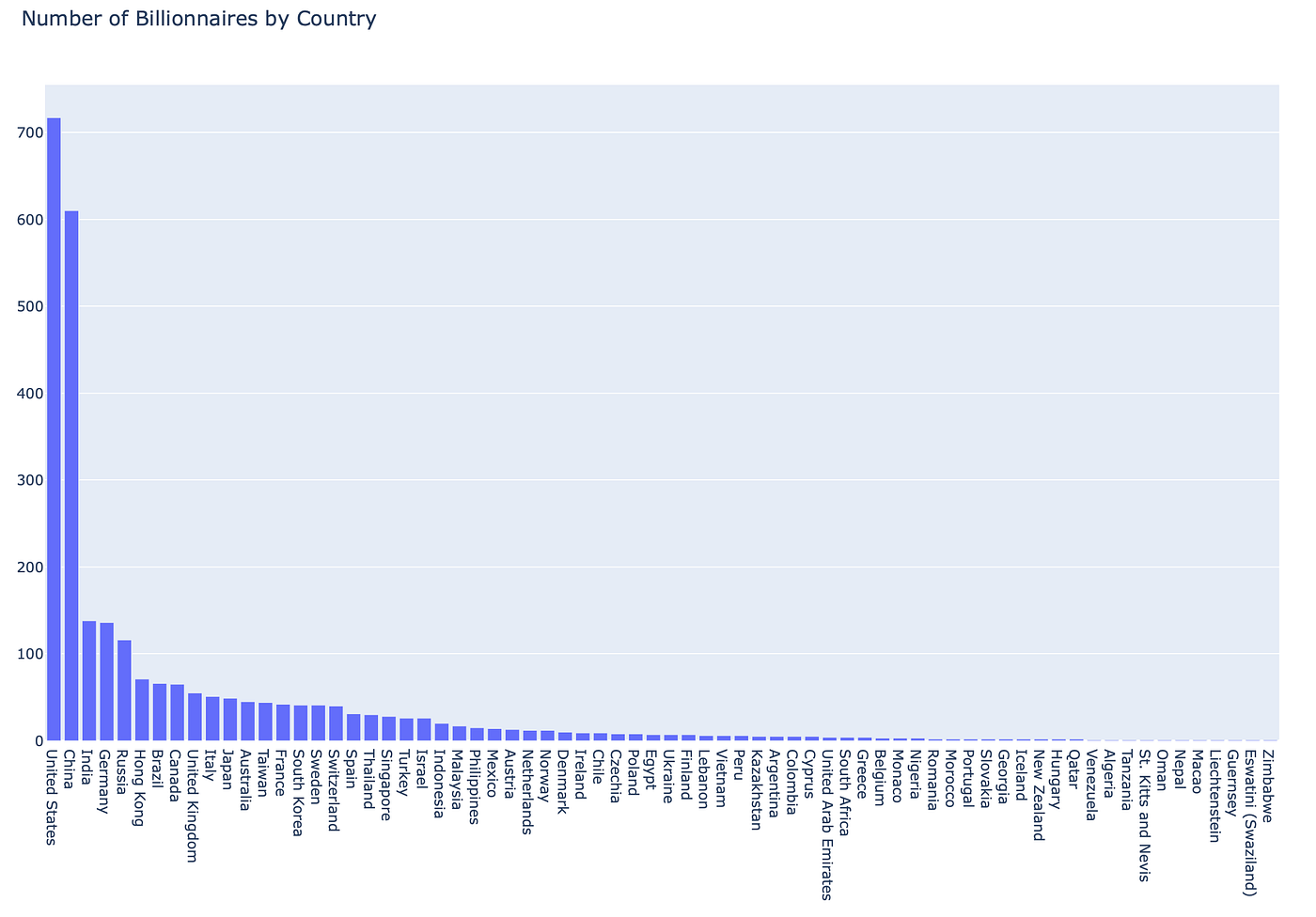Number of Billionaires by Country. Source: IDTLab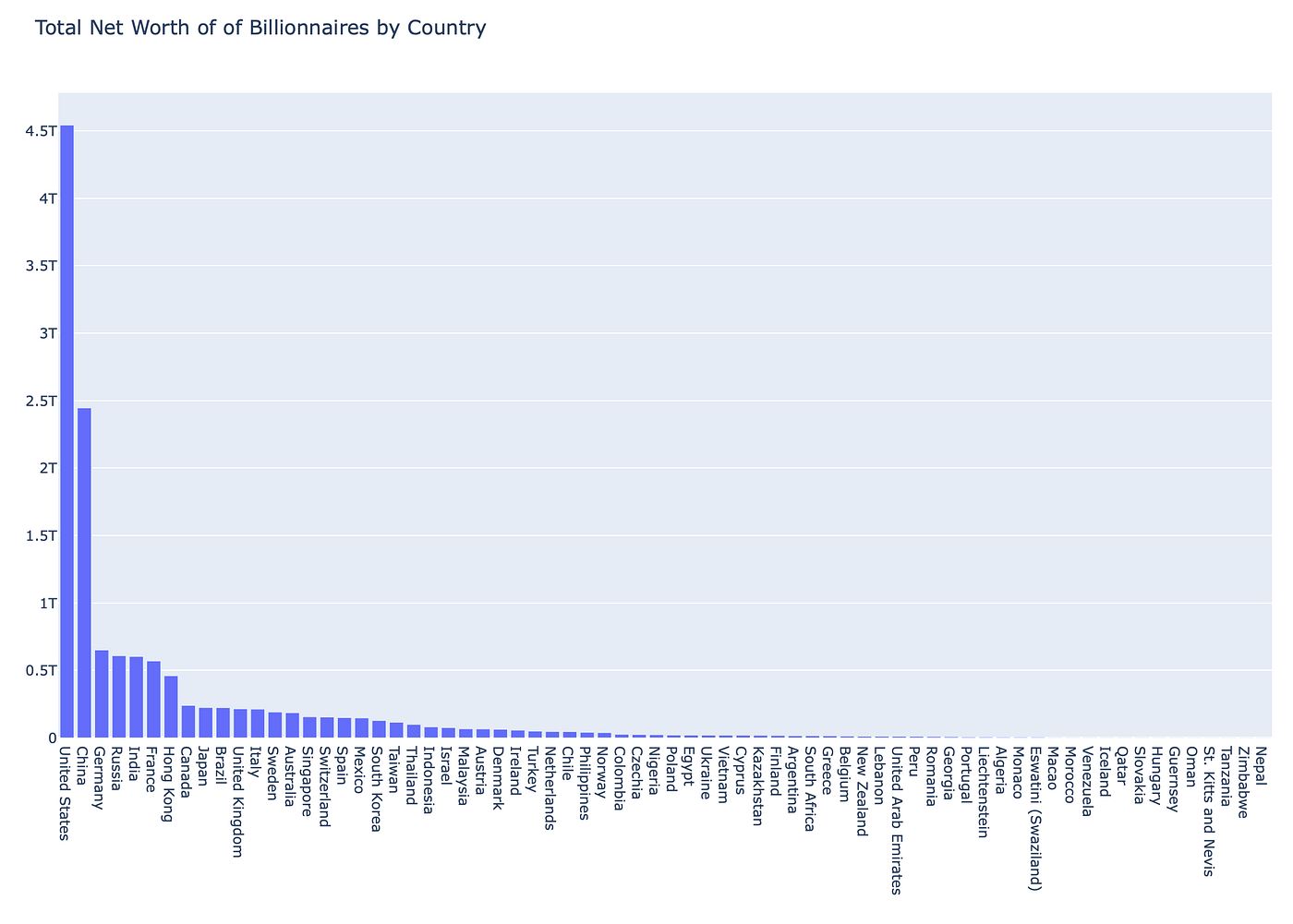Total Net Worth of Billionaires by Country. Source: IDTLab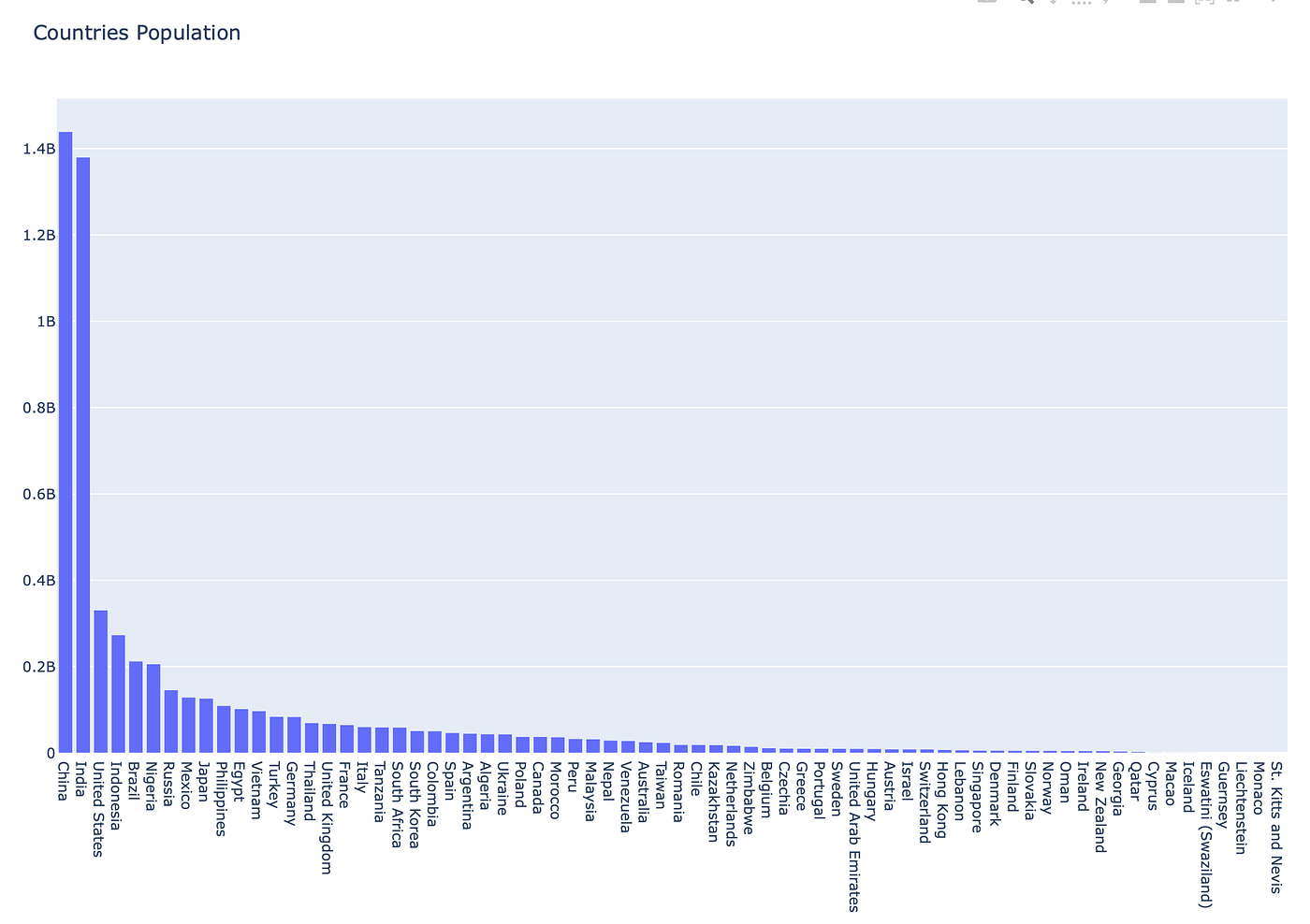Countries by Population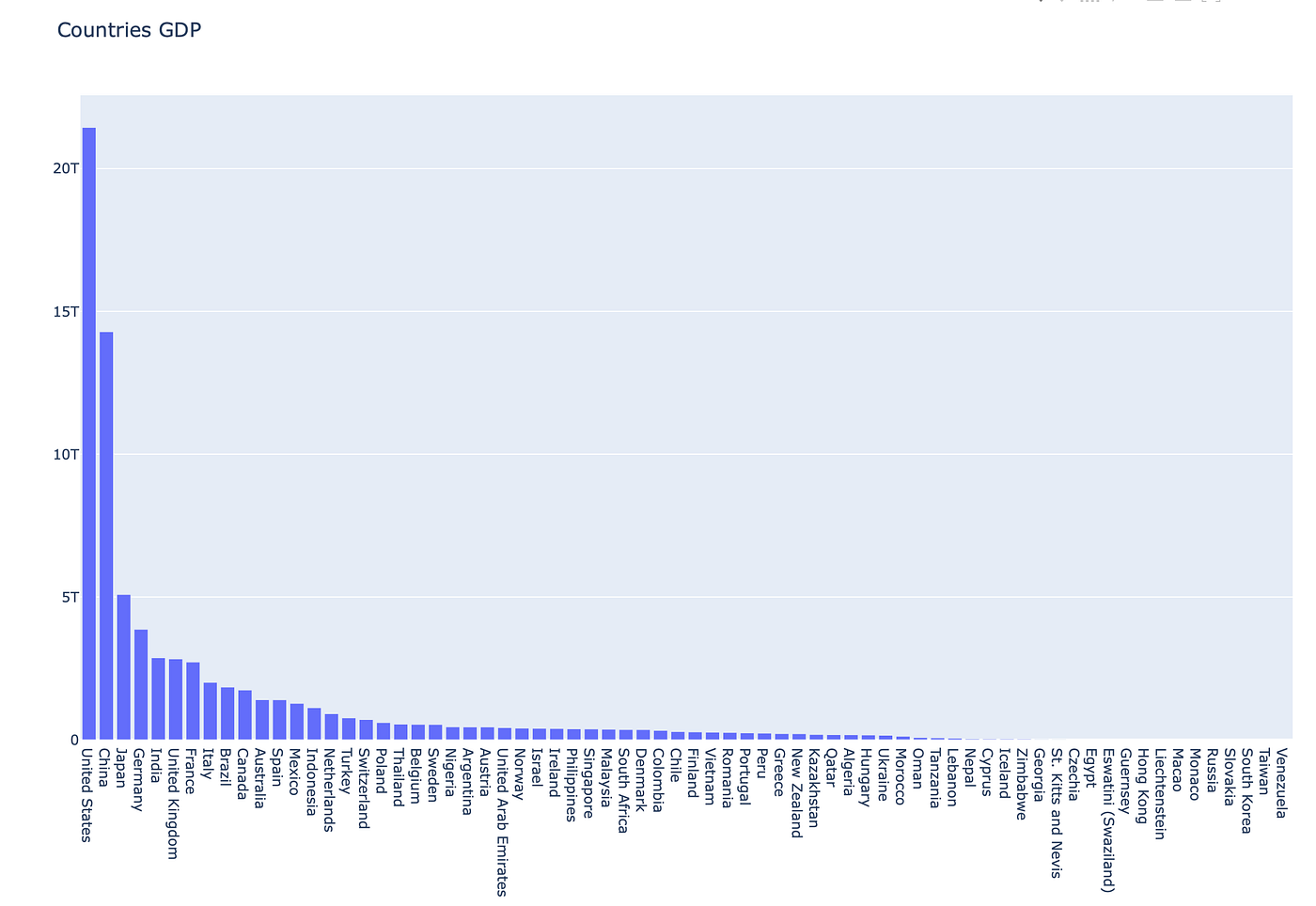Countries by Gross Domestic Product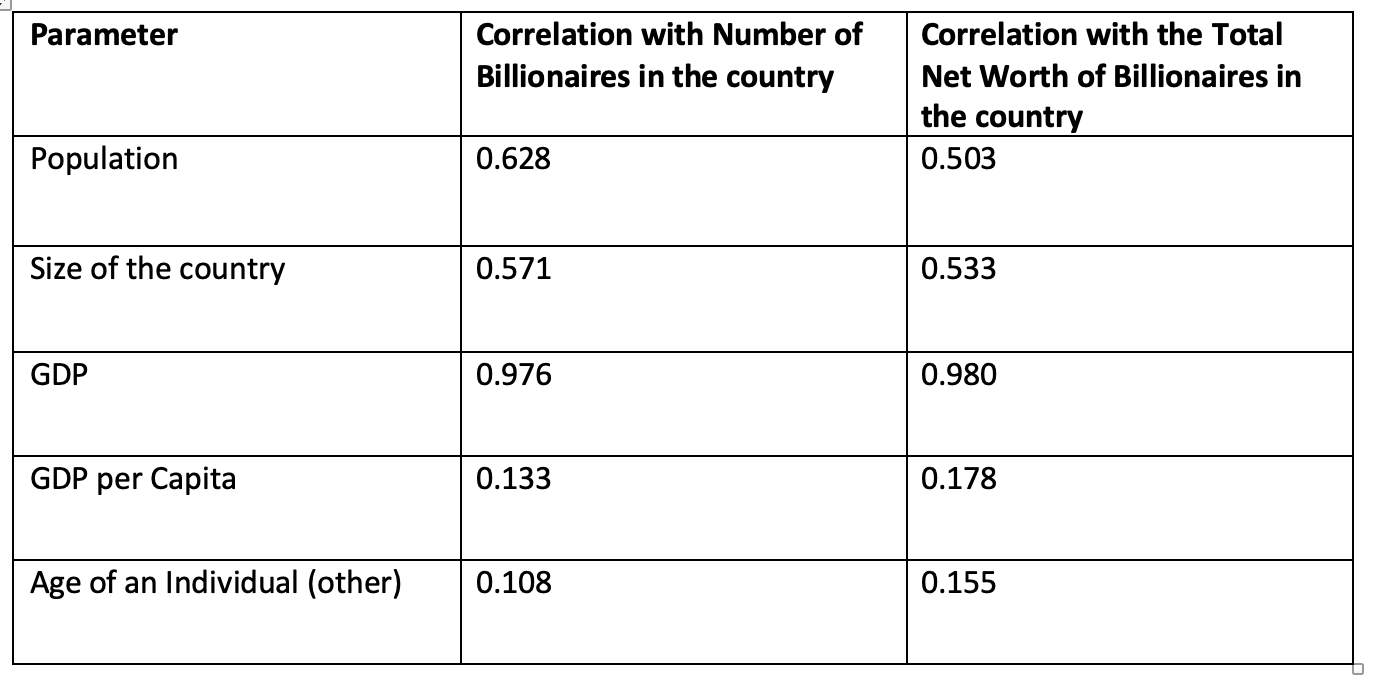Correlation of number and network of billionaires with different parameters. Source: IDTLab
1. Population of the country is only mildly significant in increasing your chances of being a billionaire while the significance is even lower in predicting how much you’d actually be worth in billions.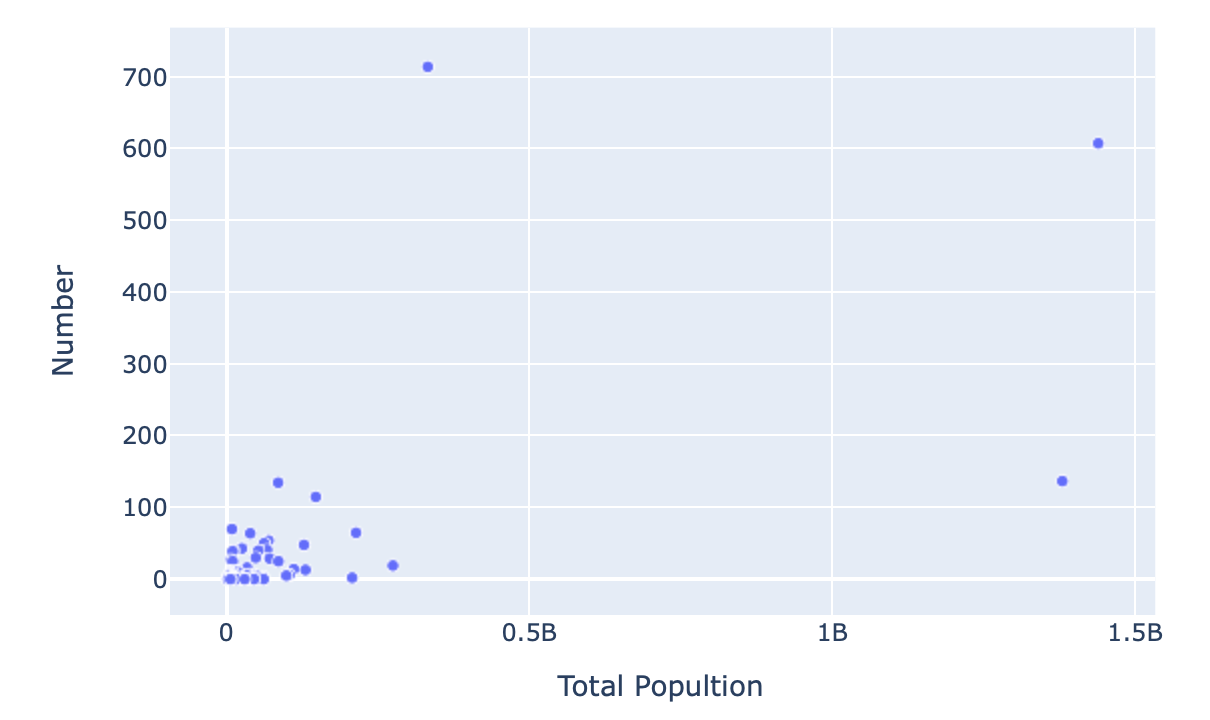Correlation Plot for Number of billionaires and population of their countries of residence. Correlation coefficient is 0.628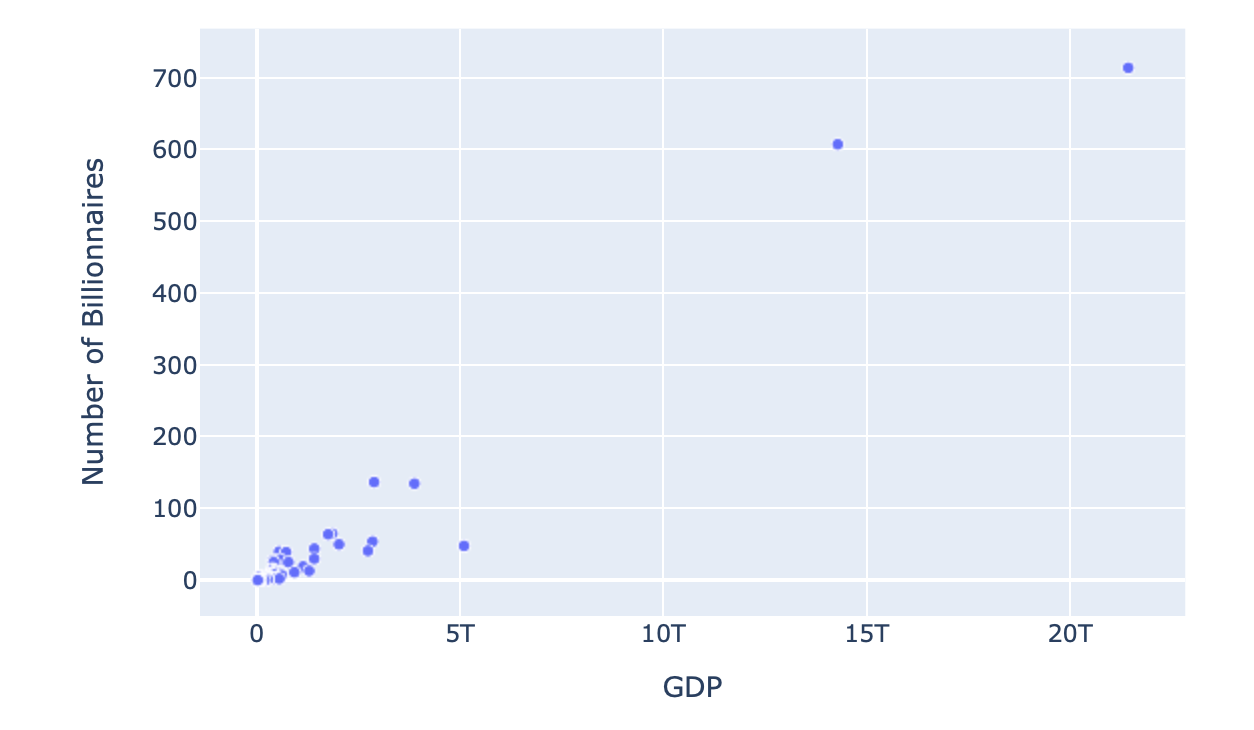Correlation plot for GDP and number of billionaires. Pearson correlation coeffieicnt = 0.976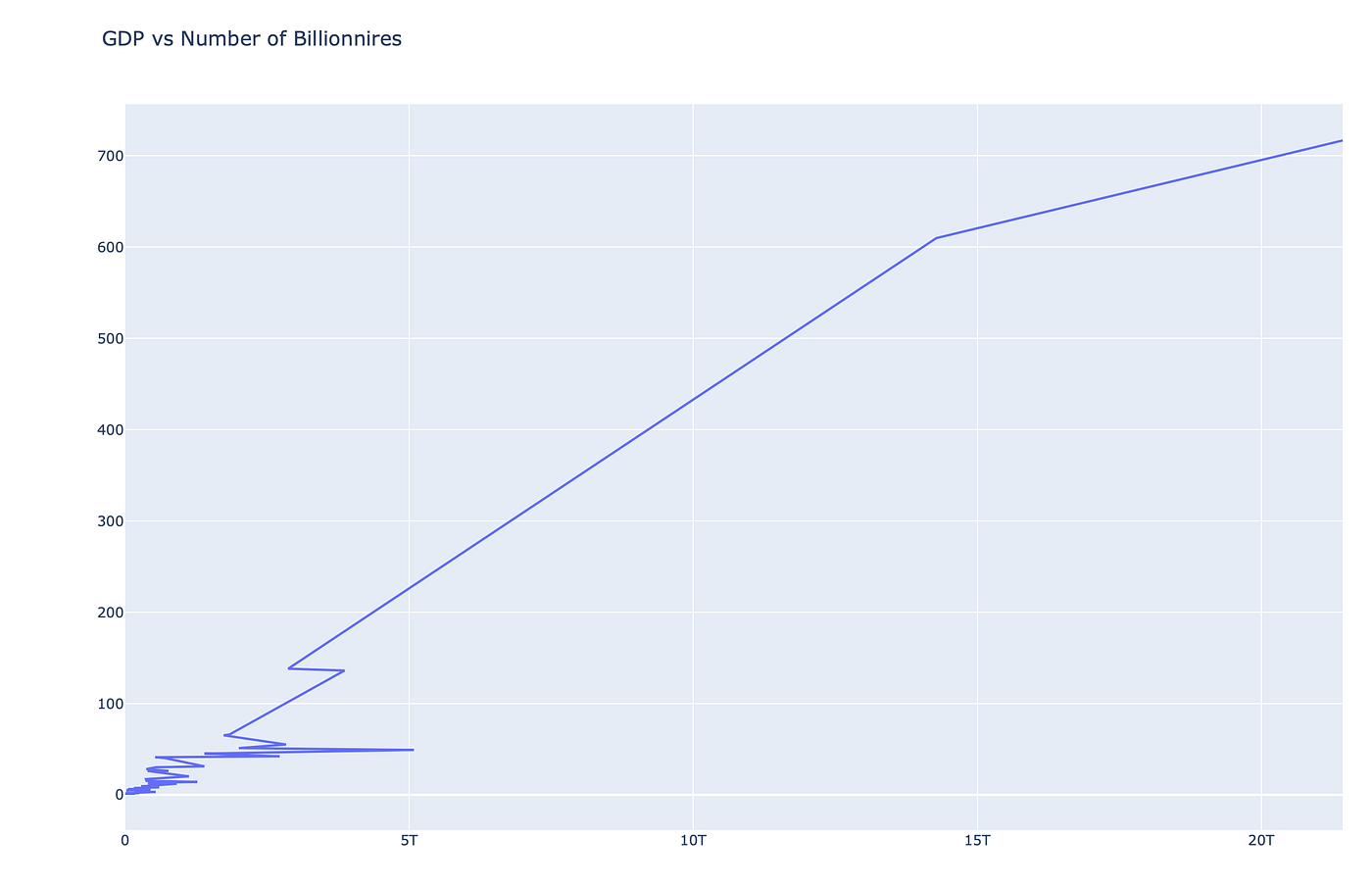Line graph for GDP of a country and number of billionaires in that country. The higher the GDP, the higher the number of ultra net worth individuals.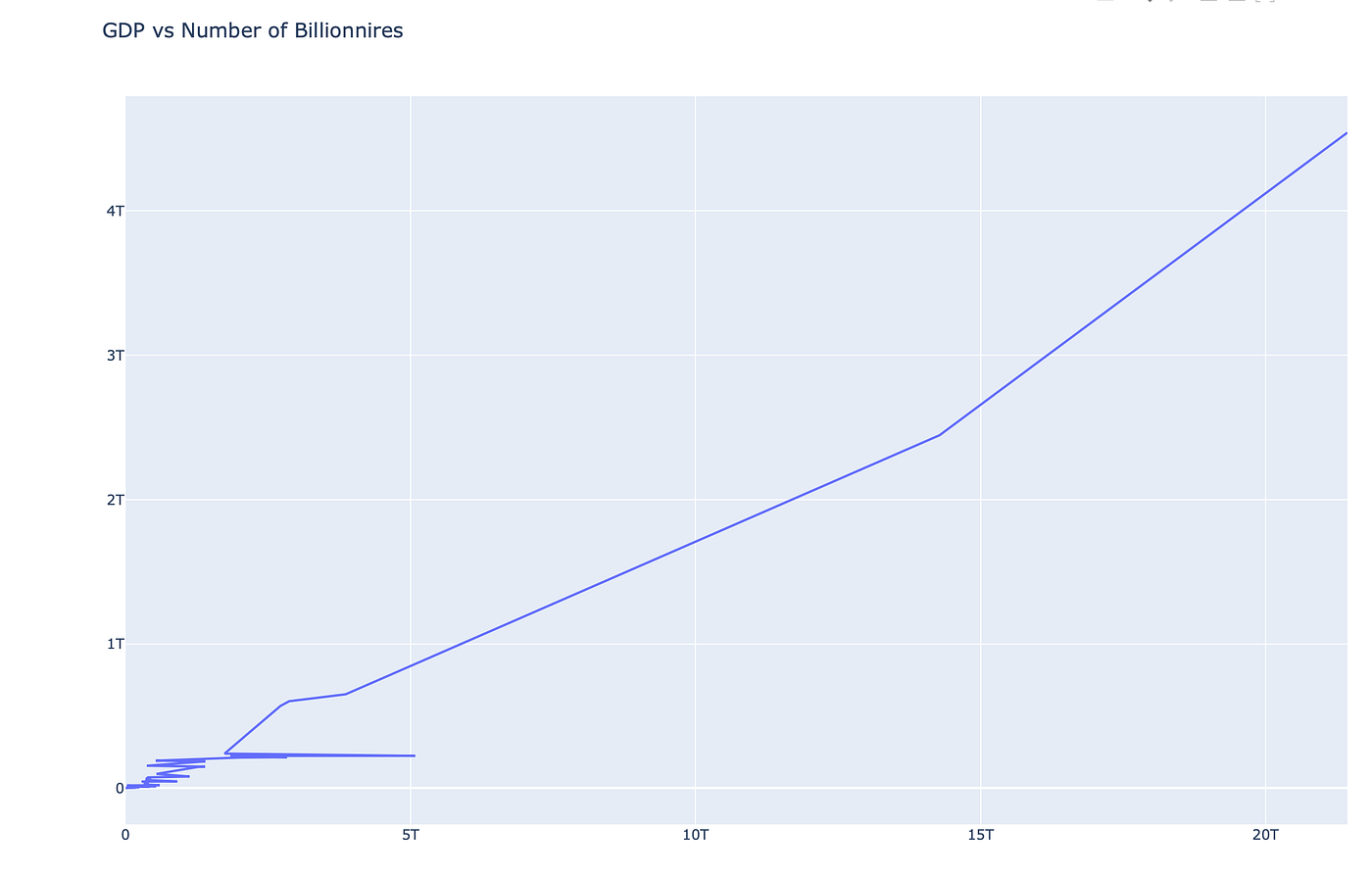Graph for GDP and total net worth of individuals from the country. Correlation coefficient = 0.98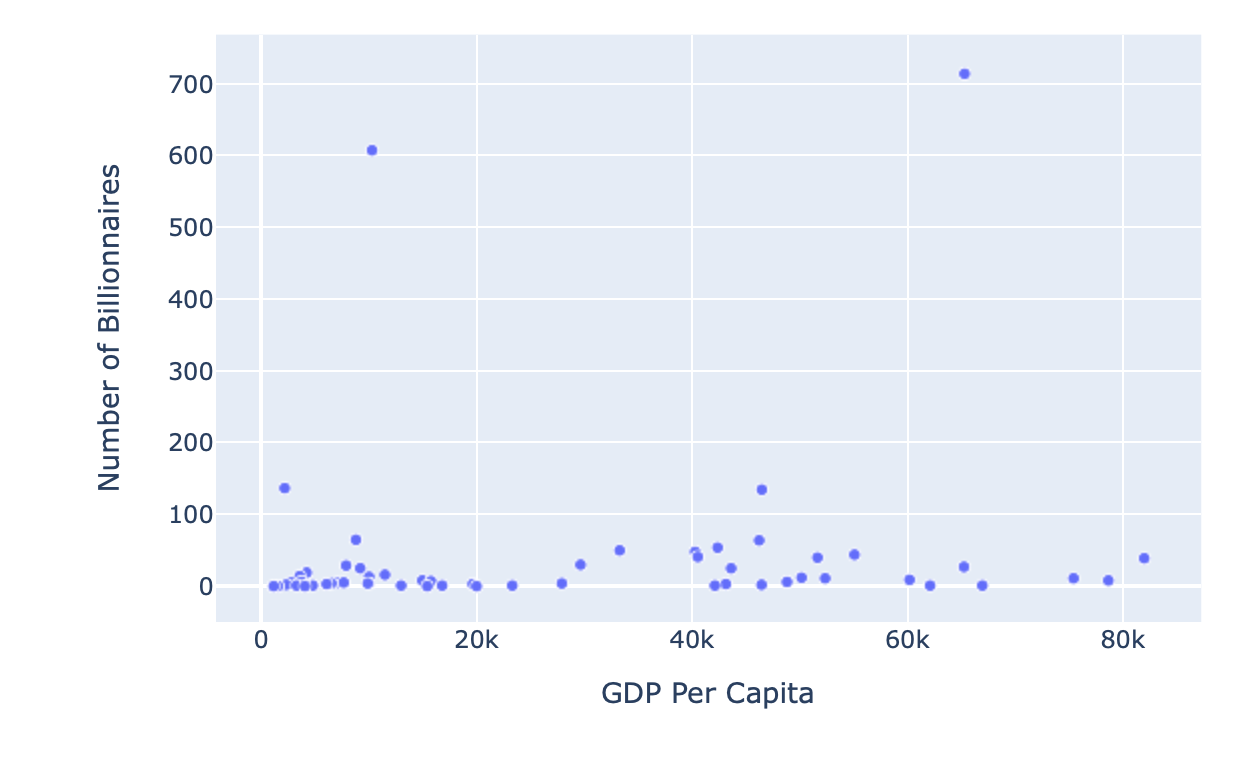No correlation between GDP per capita and the number of billionaires in that country

--

--